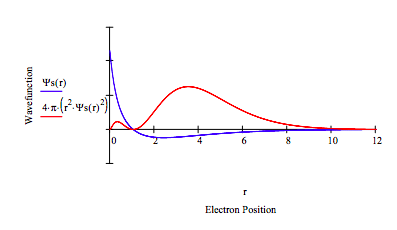# 2.34: Splitting the 2s-2p Degeneracy in the Lithium Atom

$$\newcommand{\vecs}{\overset { \rightharpoonup} {\mathbf{#1}} }$$ $$\newcommand{\vecd}{\overset{-\!-\!\rightharpoonup}{\vphantom{a}\smash {#1}}}$$$$\newcommand{\id}{\mathrm{id}}$$ $$\newcommand{\Span}{\mathrm{span}}$$ $$\newcommand{\kernel}{\mathrm{null}\,}$$ $$\newcommand{\range}{\mathrm{range}\,}$$ $$\newcommand{\RealPart}{\mathrm{Re}}$$ $$\newcommand{\ImaginaryPart}{\mathrm{Im}}$$ $$\newcommand{\Argument}{\mathrm{Arg}}$$ $$\newcommand{\norm}{\| #1 \|}$$ $$\newcommand{\inner}{\langle #1, #2 \rangle}$$ $$\newcommand{\Span}{\mathrm{span}}$$ $$\newcommand{\id}{\mathrm{id}}$$ $$\newcommand{\Span}{\mathrm{span}}$$ $$\newcommand{\kernel}{\mathrm{null}\,}$$ $$\newcommand{\range}{\mathrm{range}\,}$$ $$\newcommand{\RealPart}{\mathrm{Re}}$$ $$\newcommand{\ImaginaryPart}{\mathrm{Im}}$$ $$\newcommand{\Argument}{\mathrm{Arg}}$$ $$\newcommand{\norm}{\| #1 \|}$$ $$\newcommand{\inner}{\langle #1, #2 \rangle}$$ $$\newcommand{\Span}{\mathrm{span}}$$$$\newcommand{\AA}{\unicode[.8,0]{x212B}}$$

In lithium the ground state electronic configuration is 1s22s1. 1s22p1 is an excited state because the s-p degeneracy of the one-electron hydrogen atom has been split by the presence of the core (1s2) electrons. A simple variational calculation on Li+ using Ψ(r) = (α3/π)1/2exp(-αr) to represent the core electrons yields the following optimum for the variational parameter α = 2.6875.

We can model the behavior of the 2s electron by assuming that is attracted to the nucleus and repelled by the core electrons. The attraction for the nucleus is simply the familiar -Z/r coulombic interaction. The electrostatic interaction with the core electrons is given by,

$V_{core} = 2 \left( \frac{1 - \text{exp} (-2 \alpha \text{r}}{ \text{r}} - \alpha \text{exp} ( -2 \alpha \text{r} ) \right) \nonumber$

The next steps are to calculate the orbital energies of the 2s and 2p states by numerical integration of Schrödinger's equation. First, the 2s orbital energy.

$\begin{matrix} \text{Reduced mass:} & \mu = 1 & \text{Nuclear charge:} & \text{Z} = 3 & \text{Integration limit:} & r_{max} = 12 \\ \text{Angular momentum:} & \text{L} = 0 & \text{Energy guess:} & \text{Es} = -.1748 & \text{r} = 0,~.01 .. \text{r}_{ \text{max}} \end{matrix} \nonumber$

$\begin{matrix} \text{Given} & \frac{-1}{2 \mu} \frac{d^2}{dr^2} \Psi s(r) - \frac{1}{r \mu} \frac{d}{dr} \Psi s(r) + \left[ 2 \left( \frac{1 - \text{exp} ( -2 \alpha r}{r} - \alpha \text{exp} (-2 \alpha r ) \right) + \frac{ \text{L} ( \text{L} + 1)}{2 \mu r^2} - \frac{Z}{r} \right] \Psi s(r) = \text{Es} \Psi s (r)\end{matrix} \nonumber$

Seed values for wave function and its first derivative:

$\begin{matrix} \Psi s (.001) = 1 & \Psi (.001 ) = 0.1 \end{matrix} \nonumber$

$\Psi s = \text{Odesolve} \left( r,~ r_{max} \right) \nonumber$

Normalize the wavefunction:

$\Psi s (r) = \left( \int_0^{ r_{max}} \Psi (r)^2 4 \pi r^2 dr \right)^{-0.5} \Psi s (r) \nonumber$Setting L = 1 above demonstrates that the 2p state does not have the same energy as the 2s state. The next step is to demonstrate that the 2p energy is -0.1259 Eh.

$\begin{matrix} \text{Integration limit:} & r_{max} = 20 & \text{Angular momentum:} & L = 1 & \text{Energy guess:} & Ep = -.1259 \end{matrix} \nonumber$

$\text{Given} ~ \frac{-1}{2 \mu} \frac{d^2}{dr^2} \Psi p(r) - \frac{1}{r \mu} \frac{d}{dr} \Psi p(r) + \left[ 2 \frac{1 - \text{exp}( -2 \alpha r}{r} - \alpha \text{exp} (-2 \alpha r ) \right] \Psi p (r) = Ep \Psi p(r) \nonumber$

Seed values for wave function and its first derivative:

$\begin{matrix} \Psi p (.001) = 0 & \Psi p (.001) = 0.001 \end{matrix} \nonumber$

$\Psi p = \text{Odesolve} \left( r,~ r_{max} \right) \nonumber$

Normalize the wavefunction:

$\Psi p (r) = \left( \int_0^{r_{max}} \Psi p (r)^2 4 \pi r^2 dr \right)^{-0.5} \Psi p (r) \nonumber$

$r = 0,~.01 .. r_{max} \nonumber$Note that 2s-2p degeneracy has indeed been split. The splitting, E2p - E2s = .0488 hartree = 1.33 eV. Herzberg reports an experimental splitting of 1.85 eV. The results of this model could be improved by treating α, the core electron scale factor, as an adjustable parameter.

This page titled 2.34: Splitting the 2s-2p Degeneracy in the Lithium Atom is shared under a CC BY 4.0 license and was authored, remixed, and/or curated by Frank Rioux via source content that was edited to the style and standards of the LibreTexts platform; a detailed edit history is available upon request.